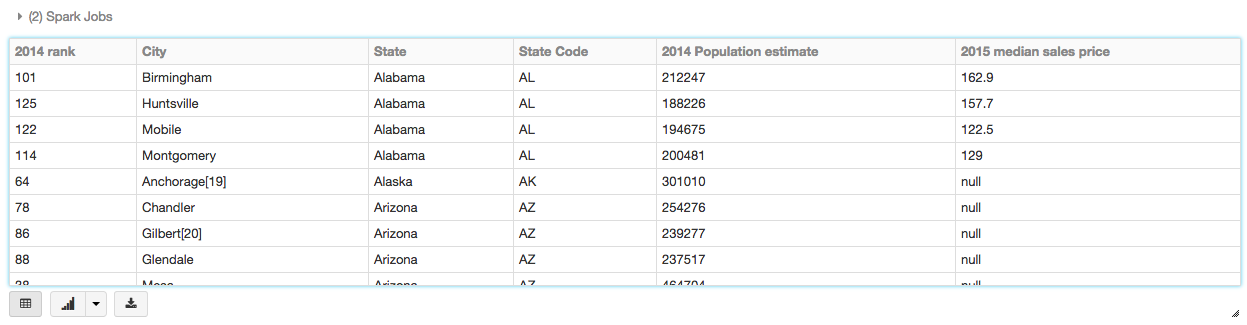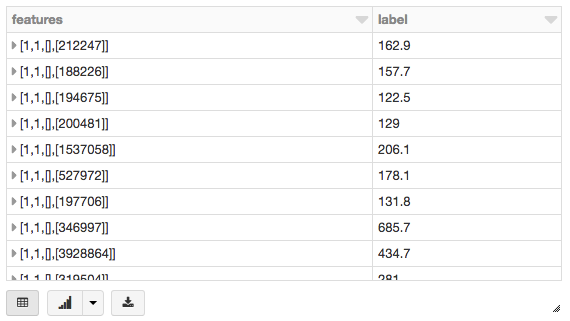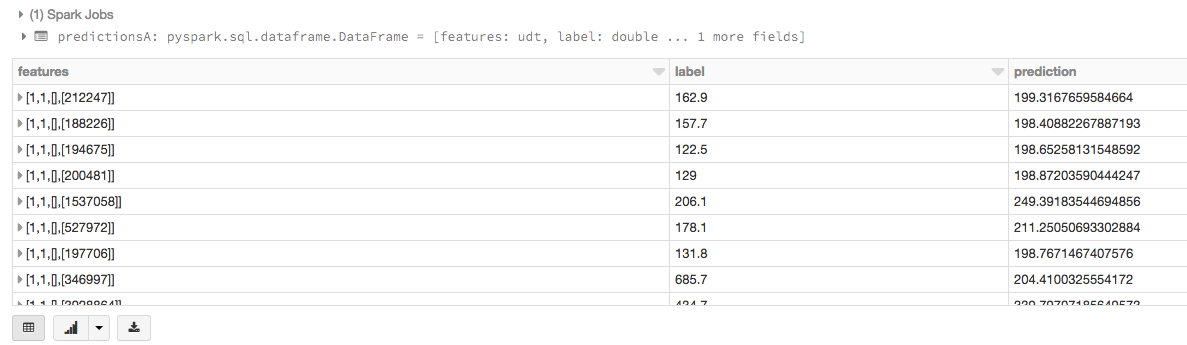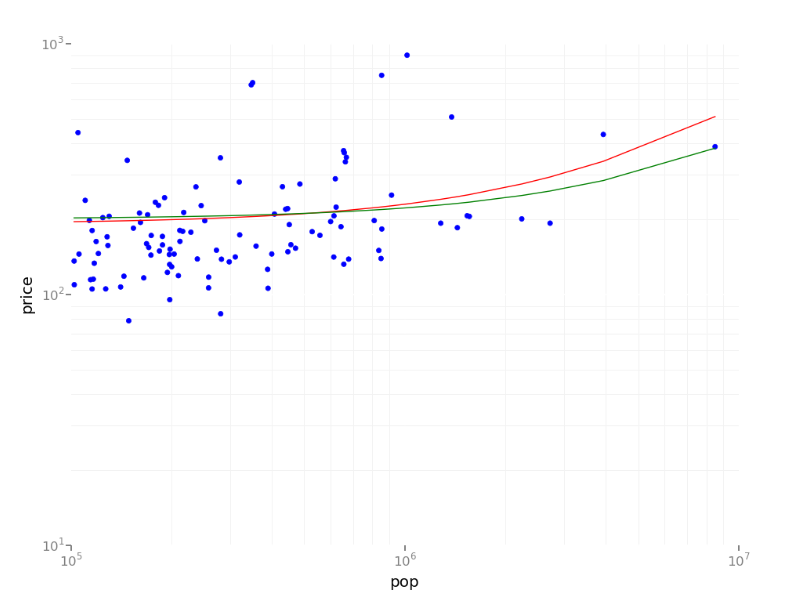Didacticiel Apache Spark™ : Démarrer avec Apache Spark sous Databricks

Vue d'ensemble

As organizations create more diverse and more user-focused data products and services, there is a growing need for machine learning, which can be used to develop personalizations, recommendations, and predictive insights. The Apache Spark machine learning library (MLlib) allows data scientists to focus on their data problems and models instead of solving the complexities surrounding distributed data (such as infrastructure, configurations, and so on).

In this tutorial module, you will learn how to:

We also provide a sample notebookthat you can import to access and run all of the code examples included in the module.

Charger l'échantillon de données

The easiest way to start working with machine learning is to use an example Databricks dataset available in the /databricks-datasetsfolder accessible within the Databricks workspace. For example, to access the file that compares city population to median sale prices of homes, you can access the file /databricks-datasets/samples/population-vs-price/data_geo.csv.

# Use the Spark CSV datasource with options specifying:
# - First line of file is a header
# - Automatically infer the schema of the data
.option("inferSchema", "true")

data.cache() # Cache data for faster reuse

To view this data in a tabular format, instead of exporting this data to a third-party tool, you can use the display() command in your Databricks notebook.

display(data)Préparer et visualiser les données pour les algorithmes de ML

In supervised learning—-such as a regression algorithm—-you typically define a label and a set of features. In this linear regression example, the label is the 2015 median sales price and the feature is the 2014 Population Estimate. That is, you use the feature (population) to predict the label (sales price).

First drop rows with missing values and rename the feature and label columns, replacing spaces with _.

data = data.dropna() # drop rows with missing values
exprs = [col(column).alias(column.replace(' ', '_')) for column in data.columns]

To simplify the creation of features, register a UDF to convert the feature (2014_Population_estimate) column vector to a VectorUDT type and apply it to the column.

from pyspark.ml.linalg import Vectors, VectorUDT

spark.udf.register("oneElementVec", lambda d: Vectors.dense([d]), returnType=VectorUDT())
tdata = data.select(*exprs).selectExpr("oneElementVec(2014_Population_estimate) as features", "2015_median_sales_price as label")

Then display the new DataFrame:

display(tdata)Exécuter le modèle de régression linéaire

In this section, you run two different linear regression models using different regularization parameters to determine how well either of these two models predict the sales price (label) based on the population (feature).

Build the model

# Import LinearRegression class
from pyspark.ml.regression import LinearRegression

# Define LinearRegression algorithm
lr = LinearRegression()

# Fit 2 models, using different regularization parameters
modelA = lr.fit(data, {lr.regParam:0.0})
modelB = lr.fit(data, {lr.regParam:100.0})

Using the model, you can also make predictions by using the transform() function, which adds a new column of predictions. For example, the code below takes the first model (modelA) and shows you both the label (original sales price) and prediction (predicted sales price) based on the features (population).

# Make predictions
predictionsA = modelA.transform(data)
display(predictionsA)Évaluer le modèle

To evaluate the regression analysis, calculate the root mean square error using the RegressionEvaluator. Here is the Python code for evaluating the two models and their output.

from pyspark.ml.evaluation import RegressionEvaluator
evaluator = RegressionEvaluator(metricName="rmse")
RMSE = evaluator.evaluate(predictionsA)
print("ModelA: Root Mean Squared Error = " + str(RMSE))
# ModelA: Root Mean Squared Error = 128.602026843

predictionsB = modelB.transform(data)
RMSE = evaluator.evaluate(predictionsB)
print("ModelB: Root Mean Squared Error = " + str(RMSE))
# ModelB: Root Mean Squared Error = 129.496300193

Visualiser le modèle

As is typical for many machine learning algorithms, you want to visualize the scatterplot. Since Databricks supports pandas and ggplot, the code below creates a linear regression plot using pandas DataFrame (pydf) and ggplot to display the scatterplot and the two regression models.

# Import numpy, pandas, and ggplot
import numpy as np
from pandas import *
from ggplot import *

# Create Python DataFrame
pop = data.map(lambda p: (p.features)).collect()
price = data.map(lambda p: (p.label)).collect()
predA = predictionsA.select("prediction").map(lambda r: r).collect()
predB = predictionsB.select("prediction").map(lambda r: r).collect()

# Create a Pandas DataFrame
pydf = DataFrame({'pop':pop,'price':price,'predA':predA, 'predB':predB})
Visualizing the Model
# Create scatter plot and two regression models (scaling exponential) using ggplot
p = ggplot(pydf, aes('pop','price')) +
geom_point(color='blue') +
geom_line(pydf, aes('pop','predA'), color='red') +
geom_line(pydf, aes('pop','predB'), color='green') +
scale_x_log10() + scale_y_log10()
display(p)We also provide a sample notebookthat you can import to access and run all of the code examples included in the module.